• 基于密度的聚类matlab程序代码 ，轻松理解密度聚类，并根据个人需求在此基础上进行改进，归为己用。
• 基于密度的聚类是聚类算法中的一种，其主要优点是可以发现任意形状的簇，但处理大数据集时效果不佳，为此提出了一种改进的算法M-DBSCAN，保留了基于密度聚类算法的优点，同时克服了以往算法不能处理大数据集的缺点。...
• I . 聚类主要算法 II . 基于划分的聚类方法 III . 基于层次的聚类方法 IV . 聚合层次聚类 图示 V ....VI . 基于层次的聚类方法 切割点选取 VII . 基于密度的方法 VIII . 基于方格的方法 IX . 基于模型的方法
文章目录I . 聚类主要算法II . 基于划分的聚类方法III . 基于层次的聚类方法IV . 聚合层次聚类 图示V . 划分层次聚类 图示VI . 基于层次的聚类方法 切割点选取VII . 基于密度的方法VIII . 基于方格的方法IX . 基于模型的方法

I . 聚类主要算法

聚类主要算法 :

① 基于划分的聚类方法 : K-Means 方法 ;
② 基于层次的聚类方法 : Birch ;
③ 基于密度的聚类方法 : DBSCAN ( Density-Based Spatial Clustering of Applications with Noise ) ;
④ 基于方格的方法 ;
⑤ 基于模型的方法 : GMM 高斯混合模型 ;

II . 基于划分的聚类方法

基于划分的方法 简介 : 基于划分的方法 , 又叫基于距离的方法 , 基于相似度的方法 ;

① 概念 : 给定 $n$ 个数据样本 , 使用划分方法 , 将数据构建成 $k$ 个划分 $(k \leq n)$ , 每个划分代表一个聚类 ;
② 分组 : 将数据集 分成 $k$ 组 , 每个分组至少要有一个样本 ;
③ 分组与样本 对应关系 : 每个分组有 $1$ 个或多个样本对象 ( $1$ 对多 ) , 每个对象同时只能在 $1$ 个分组中 ( $1$ 对 $1$ ) ;
④ 硬聚类 与 软聚类 : 每个数据对象只能属于一个组 , 这种分组称为硬聚类 ; 软聚类每个对象可以属于不同的组 ;

III . 基于层次的聚类方法

1 . 基于层次的聚类方法 概念 : 将数 据集样本对象 排列成 树结构 , 称为 聚类树 , 在指定的层次 ( 步骤 ) 上切割数据集样本 , 切割后时刻的 聚类分组 就是 聚类算法的 聚类结果 ;

2 . 基于层次的聚类方法 : 一棵树可以从叶子节点到根节点 , 也可以从根节点到叶子节点 , 基于这两种顺序 , 衍生出两种方法分支 , 分别是 : 聚合层次聚类 ,  划分层次聚类 ;

3 . 聚合层次聚类 ( 叶子节点到根节点 ) : 开始时 , 每个样本对象自己就是一个聚类 , 称为 原子聚类 , 然后根据这些样本之间的 相似性 , 将这些样本对象 ( 原子聚类 ) 进行 合并 ;
常用的聚类算法 : 大多数的基于层次聚类的方法 , 都是 聚合层次聚类 类型的 ; 这些方法从叶子节点到根节点 , 逐步合并的原理相同 ; 区别只是聚类间的相似性计算方式不同 ;

4 . 划分层次聚类 ( 根节点到叶子节点 ) :  开始时 , 整个数据集的样本在一个总的聚类中 , 然后根据样本之间的相似性 , 不停的切割 , 直到完成要求的聚类操作 ;

5 . 算法性能 : 基于层次的聚类方法的时间复杂度为 $O(N^2)$ , 如果处理的样本数量较大 , 性能存在瓶颈 ;

IV . 聚合层次聚类 图示

1 . 聚合层次聚类 图示 :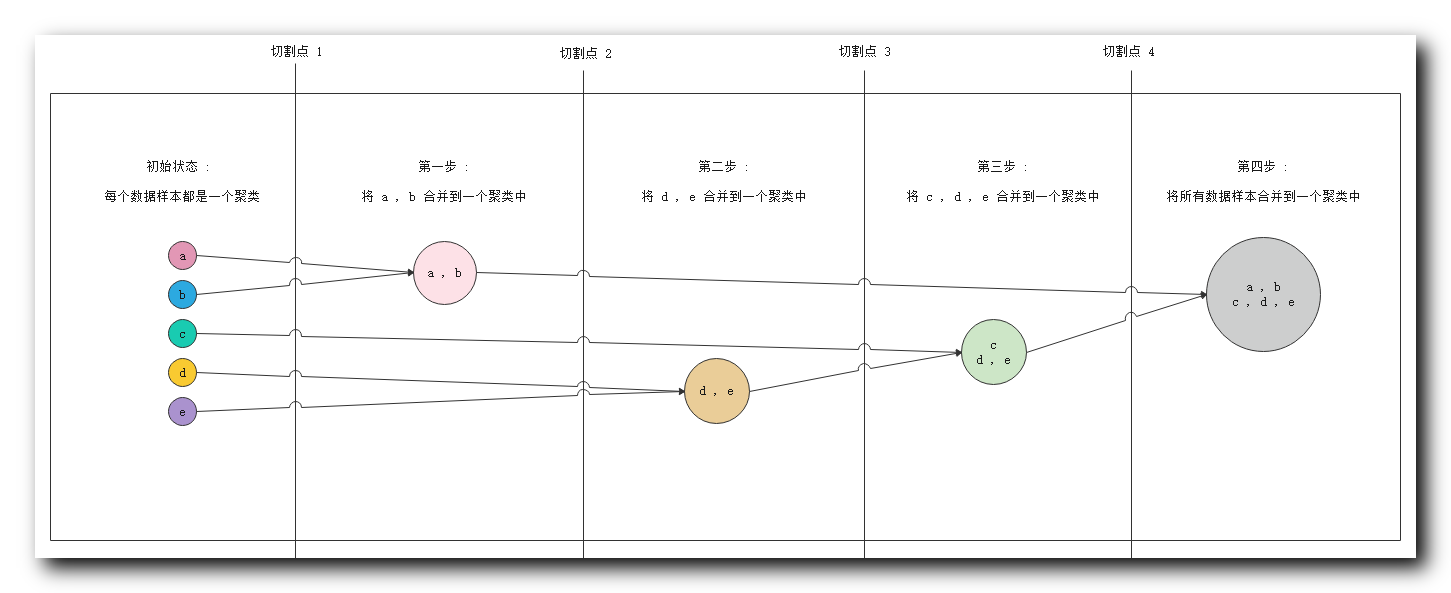① 初始状态 : 最左侧 五个 数据对象 , 每个都是一个聚类 ;
② 第一步 : 分析相似度 , 发现 $a , b$ 相似度很高 , 将 $\{a ,b\}$ 分到一个聚类中 ;
③ 第二步 : 分析相似度 , 发现 $d, e$ 相似度很高 , 将 $\{d, e\}$ 分到一个聚类中 ;
④ 第三步 : 分析相似度 , 发现 $c$ 与 $d,e$ 相似度很高 , 将 $c$ 数据放入 $\{d, e\}$ 聚类中 , 组成 $\{c,d, e\}$ 聚类 ;
⑤ 第四步 : 分析相似度 , 此时要求的相似度很低就可以将不同的样本进行聚类 , 将前几步生成的两个聚类 , 合并成一个聚类 $\{a, b, c, d, e\}$ ;

2 . 切割点说明 : 实际进行聚类分析时 , 不会将所有的步骤走完 , 这里提供四个切割点 , 聚类算法进行聚类时 , 可以在任何一个切割点停止 , 使用当前的聚类分组当做聚类结果 ;

① 切割点 $1$ : 在切割点 $1$ 停止 , 会得到 $5$ 个聚类分组 , $\{a\}$ , $\{b\}$, $\{c\}$, $\{d\}$ , $\{e\}$ ;
② 切割点 $2$ : 在切割点 $2$ 停止 , 会得到 $4$ 个聚类分组 , $\{a, b\}$ , $\{c\}$, $\{d\}$ , $\{e\}$ ;
③ 切割点 $3$ : 在切割点 $3$ 停止 , 会得到 $3$ 个聚类分组 , $\{a, b\}$ , $\{c\}$, $\{d, e\}$ ;
④ 切割点 $4$ : 在切割点 $4$ 停止 , 会得到 $2$ 个聚类分组 ; $\{a, b\}$ , $\{c, d, e\}$ ;
⑤ 走完整个流程 : 会得到 $1$ 个聚类分组 ,  $\{a, b ,c, d, e\}$ ;

V . 划分层次聚类 图示

1 . 划分层次聚类 图示 :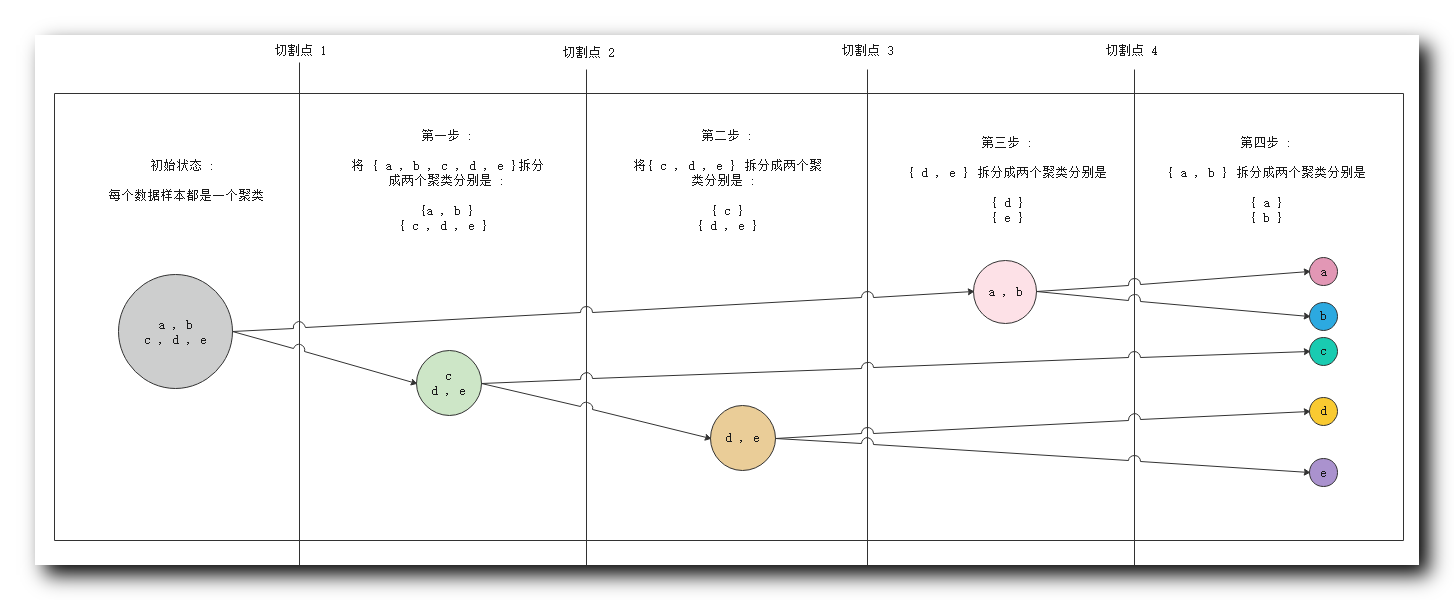① 初始状态 : 最左侧 五个 数据对象 , 属于一个聚类 ;
② 第一步 : 分析相似度 , 切割聚类 , 将 $\{c,d, e\}$ 与 $\{a ,b\}$ 划分成两个聚类 ;
③ 第二步 : 分析相似度 , 将 $\{c,d, e\}$ 中的 $\{c\}$ 与 $\{d, e\}$ 划分成两个聚类 ;
④ 第三步 : 分析相似度 , 将 $\{d, e\}$ 拆分成 $\{d\}$ 和 $\{e\}$ 两个聚类 ;
⑤ 第四步 : 分析相似度 , 将 $\{a ,b\}$ 拆分成 $\{a\}$ 和 $\{b\}$ 两个聚类 , 至此所有的数据对象都划分成了单独的聚类 ;

2 . 切割点说明 : 实际进行聚类分析时 , 不会将所有的步骤走完 , 这里提供四个切割点 , 聚类算法进行聚类时 , 可以在任何一个切割点停止 , 使用当前的聚类分组当做聚类结果 ;

① 切割点 $1$ : 在切割点 $1$ 停止 , 会得到 $1$ 个聚类分组 ,  $\{a, b ,c, d, e\}$ ;
② 切割点 $2$ : 在切割点 $2$ 停止 , 会得到 $2$ 个聚类分组 ; $\{a, b\}$ , $\{c, d, e\}$ ;
③ 切割点 $3$ : 在切割点 $3$ 停止 , 会得到 $3$ 个聚类分组 , $\{a, b\}$ , $\{c\}$, $\{d, e\}$\$ ;
④ 切割点 $4$ : 在切割点 $4$ 停止 , 会得到 $4$ 个聚类分组 , $\{a, b\}$ , $\{c\}$, $\{d\}$ , $\{e\}$ ;
⑤ 走完整个流程 : 会得到 $5$ 个聚类分组 , $\{a\}$ , $\{b\}$, $\{c\}$, $\{d\}$ , $\{e\}$ ;

VI . 基于层次的聚类方法 切割点选取

1 . 算法终止条件 ( 切割点 ) : 用户可以指定聚类操作的算法终止条件 , 即上面图示中的切割点 , 如 :

① 聚类的最低个数 :  聚合层次聚类中 , $n$ 个样本 , 开始有 $n$ 个聚类 , 逐步合并 , 聚类个数逐渐减少 , 当聚类个数达到最低值 $min$ , 停止聚类算法 ;
② 聚类最高个数 : 划分层次聚类中 , $n$ 个样本 , 开始有 $1$ 个聚类 , 逐步划分 , 聚类个数逐渐增加 , 当聚类个数达到最大值 $max$ , 停止聚类算法 ;
③ 聚类样本的最低半径 : 聚类的数据样本范围不能无限扩大 , 指定一个阈值 , 只有将该阈值内的样本放入一组 ; 半径指的是所有对象距离其平均点的距离 ;

2 . 切割点回退问题 : 切割点一旦确定 , 便无法回退 ; 这里以聚合层次聚类为例 :

① 处于切割点 $4$ : 如已经执行到了步骤三 , 此时处于切割点 $4$ ,  聚类分组为 $\{a, b\}$ , $\{c, d, e\}$ ;
② 试图回退到 切割点 $3$ : 想要会回退到切割点 $3$ 的状态 , 视图将聚类分组恢复成 $\{a, b\}$ , $\{c\}$, $\{d, e\}$ ;
③ 无法回退 : 该操作是无法实现的 , 聚类分组一旦 合并 或 分裂 , 此时就无法回退 ;

VII . 基于密度的方法

1 . 基于距离聚类的缺陷 : 很多的聚类方法 , 都是 基于样本对象之间的距离 ( 相似度 ) 进行的 , 这种方法对于任意形状的分组 , 就无法识别了 , 如下图左侧的聚类模式 ;  这种情况下可以使用基于密度的方法进行聚类操作 ;
基于距离的方法 , 是基于欧几里得距离函数得来 , 其基本的形状都是球状 , 或凸形状 , 如下图右侧的形状 ; 无法计算出凹形状 , 如下图左侧的形状 ;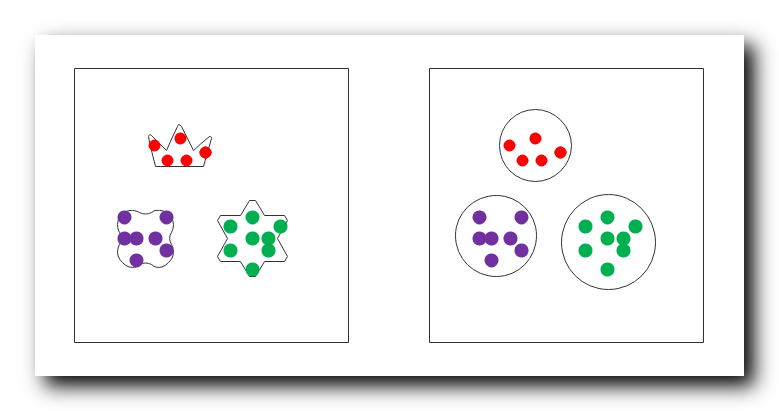2 . 基于密度的聚类方法 : 相邻的区域内 样本对象 的密度超过某个阈值 , 聚类算法就继续执行 , 如果周围区域密度都很小 , 那么停止聚类方法 ;

① 密度 : 某 单位大小 区域内的样本对象个数 ;
② 聚类分组要求 : 在聚类分组中 , 每个分组的数据样本密度都 必须达到密度要求的最低阈值 ;

3 . 基于密度的聚类方法 算法优点 :

① 排除干扰 :  过滤噪音数据 , 即密度很小 , 样本分布稀疏的数据 ;
② 增加聚类模式复杂度 : 聚类算法可以识别任意形状的分布模式 , 如上图左侧的聚类分组模式 ;

VIII . 基于方格的方法

1 . 基于方格的方法 : 将数据空间划分成 一个个方格 , 在这些方格数据结构上 , 将每个方格中的数据样本 , 当做一个数据处理 , 进行聚类操作 ;

2 . 基于方格的方法优点 : 处理速度很快 , 将每个方格都作为一个数据 , 如果分成 少数的几个方格进行聚类操作 , 聚类瞬间完成 ; 其速度与数据集样本个数无关 , 与划分的数据方格个数有关 ;

3 . 局限性 : 该方法的错误率很高 ;

IX . 基于模型的方法

基于模型的方法

① 基于统计的方法  : GMM 高斯混合模型 ;
② 神经网络方法 ;


展开全文基于划分的聚类 基于层次的聚类 基于模型的聚类
• 与基于距离的聚类算法不同的是，基于距离的聚类算法的聚类结果是球状的簇，而基于密度的聚类算法可以发现任意形状的聚类，这对于带有噪音点的数据起着重要的作用。 DBSCAN(Density-Based Spatial Clustering of...
基于距离的聚类算法，如K-Means算法。

基于密度的聚类算法主要的目标是寻找被低密度区域分离的高密度区域。
与基于距离的聚类算法不同的是，基于距离的聚类算法的聚类结果是球状的簇，而基于密度的聚类算法可以发现任意形状的聚类，这对于带有噪音点的数据起着重要的作用。

DBSCAN(Density-Based Spatial Clustering of Application with Noise

，具有噪声的基于密度的聚类方法)
经典密度聚类算法
将数据点分为以下三类：

核心点。在半径Eps内含有超过MinPts数目的点边界点。在半径Eps内点的数量小于MinPts，但是落在核心点的邻域内噪音点。既不是核心点也不是边界点的点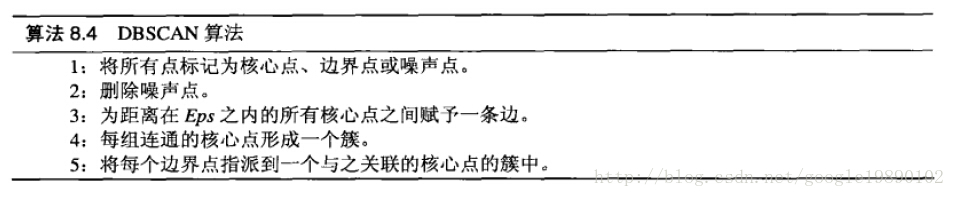展开全文• DeBaCl, 基于密度的聚类( DeBaCl ) DeBaCl: 基于密度的聚类 DeBaCl是一个基于密度的python 库，带有级别的树 。水平集树是表示概率密度函数拓扑的一种统计方法。 这里表示形式对于统计中的几个核心任务尤其有用：...
•   DBSCAN（Density-Based Spatial Clustering of Applications with Noise，具有噪声的基于密度的聚类方法）是一种基于密度的空间聚类算法 可以在有噪音的数据中发现各种形状和各种大小的簇。 寻找被低密度区域...
一、DBSCAN算法介绍
DBSCAN（Density-Based Spatial Clustering of Applications with Noise，具有噪声的基于密度的聚类方法）是一种基于密度的空间聚类算法

可以在有噪音的数据中发现各种形状和各种大小的簇。
寻找被低密度区域分离的高密度区域，这些高密度区域就是一个一个的簇，这里的密度指的是一个样本点的领域半径内包含的点的数目
可以用来过滤噪声孤立点数据，发现任意形状的簇（因为它将簇定义为密度相连的点的最大集合）
与k-means算法的不同之处在于它不需要事先指定划分的簇的数目。

二、基本概念

$\varepsilon$邻域：对于任意样本i和给定距离$\varepsilon$，样本i的$\varepsilon$邻域是指所有与样本i距离不大于$\varepsilon$的样本集合；
核心点：若样本i的$\varepsilon$邻域中至少包含MinPts个样本，则i是一个核心对象；
边界点：如果一个对象i是非核心对象，但它的领域中有核心对象，则为边界点。
噪声点：除了核心点和边界点的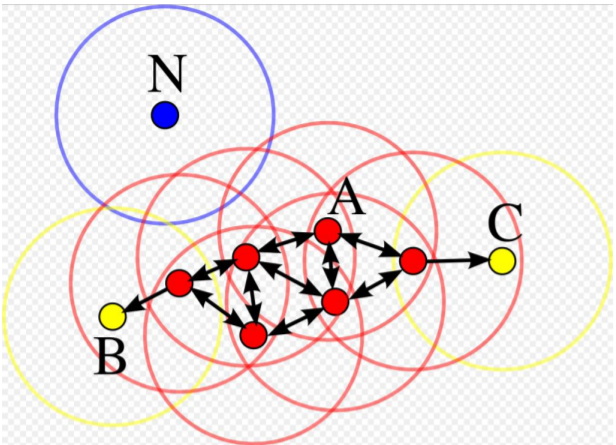密度直达：若样本m在样本p的$\varepsilon$邻域中，且p是核心对象，则称样本m由样本p密度直达
密度可达：对于样本i和样本j，如果存在样本序列p1，p2，…，pn，其中p1=i，pn=j，并且pm由pm-1密度直达，则称样本i与样本j密度可达；如p与q可达
密度相连：对于样本s和样本r，若存在样本o使得s与r均由0密度可达，则称s与r密度相连。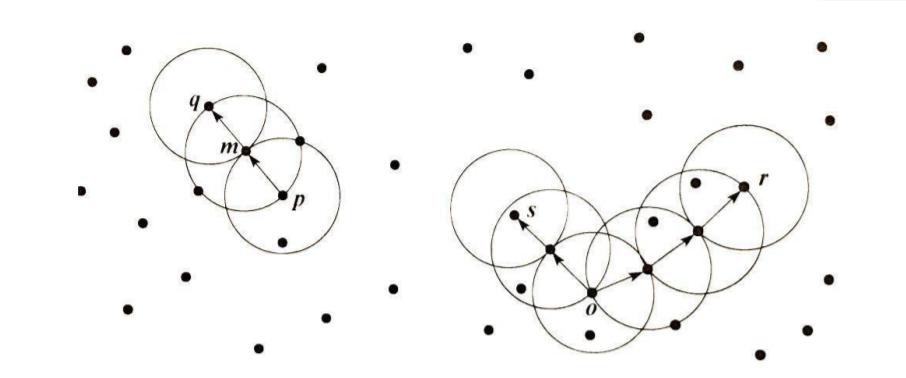三、优缺点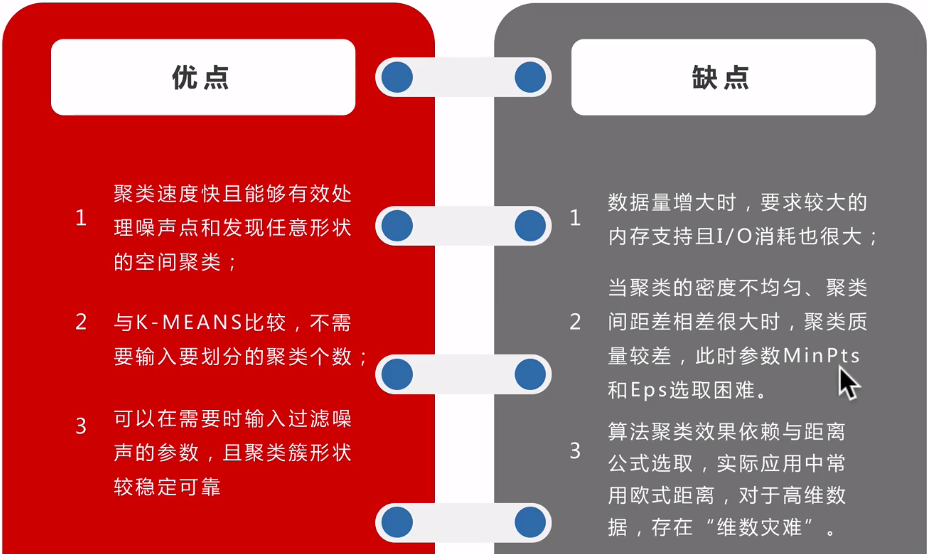如下图， eps, MinPoints选取不同，结果会有很大的影响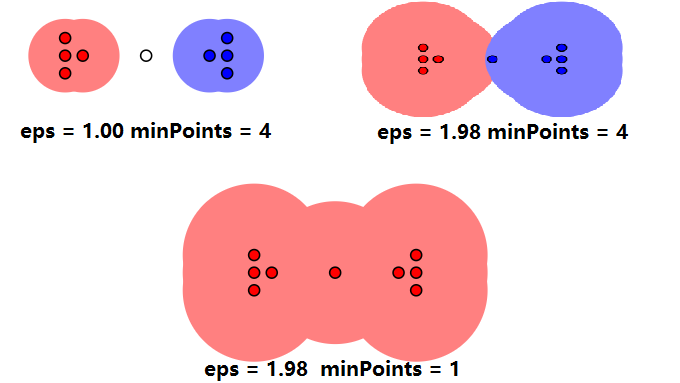3.3、瓶颈
在 DBSCAN 算法中，由于边界点可以被不止一个簇密度相连，对数据不同的处理顺序可能会导致不同的处理结果，所以不确定性是 DBSCAN 的问题之一。DBSCAN 的聚类效果会受到欧式距离的通病维数灾难的影响，与此同时对于在密度上有较大差异的数据，最小样本个数 MinPts 的选取又非常困难。所以如何选择邻域距离e和邻域最小样本个数 MinPts 是DBSCAN 算法中非常关键的问题。
Reference

DBSCAN算法学习笔记及scala实现


展开全文• 基于密度的聚类算法 DBscan 基于密度的聚类算法的结果是球状的簇 基于密度的聚类算法的结果可以是任意形状，这有利于处理带有噪音点的数据 DBscan 相关概念 点P的邻接半径 eps : 以点P为中心，以 eps 为半径...
基于密度的聚类算法 DBscan

基于密度的聚类算法的结果是球状的簇

基于密度的聚类算法的结果可以是任意形状，这有利于处理带有噪音点的数据


DBscan 相关概念

点P的邻接半径 eps : 以点P为中心，以 eps 为半径
点P的eps邻域 ： 与点P的距离 <= eps的所有点的集合
密度域值 minPts ：指定的一个数，表示最小的点的个数，它刻画了最小的密度情况，过滤掉密度稀疏的点
核心点 ： 点 P 的eps 领域点的个数 >= minPts ，则 P 称为核心点
边界点 ：点 Q 的eps 领域点的个数 < minPts ，但是 Q 落在某个核心点 P 的eps邻域内，则点 Q 称为边界点
噪音点 ：点 R 既不是核心点也不是边界点，则 R 称为噪音点
直接密度可达 ： 点 q 在点 p 的 eps 邻域内，则称 q 从 p 出发是直接密度可达
密度可达 ： 对于对象链 P1,P2,……,Pn , 若 Pi+ z1 从 Pi 出发直接密度可达，则 Pn 从 P1 出发密度可达（传递性，间接密度可达）
DBscan 算法思想

判断两点之间是否直接密度可达

def eps_neighborhood(a, b, eps):
return dist(a, b) < eps

求某点的 eps 邻域

def region_query(dataSet, point_id, eps):
n_points = dataSet.shape #shape函数是numpy.core.fromnumeric中的函数，它的功能是读取矩阵的长度
seeds = []
for i in range(0, n_points):
if eps_neighborhood(dataSet[:, point_id], dataSet[:, i], eps):
seeds.append(i)
return seeds


为核心对象聚类并合并

 合并两个存在密度相连的元素的集合


def expand_cluster(dataSet, clusterResults, point_id, cluster_id, eps, minPts):

seeds = region_query(dataSet, point_id, eps)
if len(seeds) < minPts:
clusterResults[point_id] = NOISE #标为噪音点
return False
else:
clusterResults[point_id] = cluster_id #划分到该簇
for seed_id in seeds:
clusterResults[seed_id] = cluster_id #该点的eps邻域也划分到该簇

while len(seeds) > 0: # 持续扩张，seeds里面的点的eps邻域一定与当前簇有密度可达的点，合并簇
current_point = seeds
expand_seeds = region_query(dataSet, current_point, eps)
if len(expand_seeds) >= minPts: #如果 current_point是核心点
for expand_id in range(0, len(expand_seeds)):
result_point = expand_seeds[expand_id]
if clusterResults[result_point] == UNCLASSIFIED: #未分类的类做我的seed
seeds.append(result_point)
clusterResults[result_point] == cluster_id
elif clusterResults[result_point] == NOISE: #已分类的类与我合并
clusterResults[result_point] == cluster_id
seeds = seeds[1:]
return True

DBscan输出聚类结果

def dbscan(dataSet, eps, minPts):
cluster_id = 1
n_points = dataSet.shape
clusterResults = [UNCLASSIFIED] * n_points
for point_id in range(0, n_points):
point = dataSet[:, point_id]
if clusterResults[point_id] == UNCLASSIFIED:
if expand_cluster(dataSet, clusterResults, point_id, cluster_id, eps, minPts):
cluster_id = cluster_id + 1
return clusterResults, cluster_id - 1
展开全文dbscan算法
• 基于密度的聚类-DBSCAN、OPTICS、DENCLUE，自己整理，容易理解、并举例演示算法过程，简洁明了
• 简单易学的机器学习算法—基于密度的聚类算法DBSCAN 一、基于密度的聚类算法的概述 我想了解下基于密度的聚类算法，熟悉下基于密度的聚类算法与基于距离的聚类算法，如K-Means算法之间的区别。 基于密度的聚类...
• 一、基于密度的聚类算法的概述 最近在Science上的一篇基于密度的聚类算法《Clustering by fast search and find of density peaks》引起了大家的关注(在我的博文“论文中的机器学习算法——基于密度峰值的聚类算法...
• 一、基于密度的聚类算法的概述  最近在Science上的一篇基于密度的聚类算法《Clustering by fast search and find of density peaks》引起了大家的关注(在我的博文“机器学习算法（聚类算法）-基于密度峰值的聚类...
• 本文介绍一种基于密度的聚类方法，可以聚类任意的形状。  基于密度的聚类是根据样本的密度分布来进行聚类。通常情况下，密度聚类从样本密度的角度出来，来考查样本之间的可连接性，并基于可连接样本不断扩展聚类簇...
• 机器学习 聚类篇——python实现DBSCAN（基于密度的聚类方法）摘要python实现代码计算实例 摘要 DBSCAN（Density-Based Spatial Clustering of Applications with Noise） 为一种基于密度的聚类算法，它不仅可以找出...python 无监督学习 机器学习 数据挖掘
• DBSCAN(Density-Based Spatial Clustering of Applications with Noise)是一个比较有代表性的基于密度的聚类算法。与划分和层次聚类方法不同，它将簇定义为密度相连的点的最大集合，能够把具有足够高密度的区域划分...算法 python
• I . K-Means 算法在实际应用中的缺陷 II . K-Means 初始中心点选择不恰当 ... 基于密度的聚类方法 V . 基于密度的聚类方法 DBSCAN 方法 VI . ε-邻域 VII . 核心对象 VIII . 直接密度可达 IX . 密度可达 X . 密度连接K-Means DBSCAN
• DBSCAN(Density-Based Spatial Clustering of Applications with Noise)是一个比较有代表性的基于密度的聚类算法。与划分和层次聚类方法不同，它将簇定义为密度相连的点的最大集合，能够把具有足够高密度的区域划分...
•  DBSCAN(Density-Based Spatial Clustering of Applications with Noise)是一个比较有代表性的基于密度的聚类算法。与划分和层次聚类方法不同，它将簇定义为密度相连的点的最大集合，能够把具有足够高密度的区域...机器学习
• **DBSCAN算法和实现——DBSCAN(Density-Based Spatial Clustering of Applications with Noise)是一个比较有代表性的基于密度的聚类算法，它是一种适应性极强的聚类算法，不同于K-means聚类，它无需确定簇的数量k，...
• 聚类分析（五）——基于密度的聚类算法OPTICS
• 基于密度的聚类是根据样本的密度分布来进行聚类。通常情况下，密度聚类从样本密度的角度出来，来考查样本之间的可连接性，并基于可连接样本不断扩展聚类簇，以获得最终的聚类结果。其中最著名的算法就是 DBSCAN ......